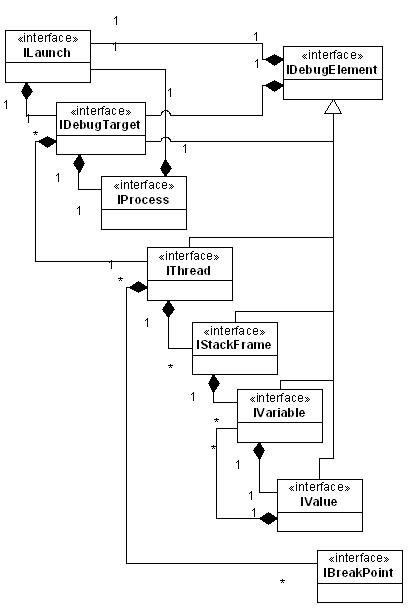## Wednesday, January 21, 2009

### Mutual recursion fun with Eclipse Debug model

I rarely come across real-world data structures which are inherently mutually recursive (a.k.a. Daisy chain recursion in CS literature). Recently, while writing a plug-in related to eclipse.debug, I came across this interesting data structure.Source Eclipse.org

Observe the IVariable ←→ IValue relationship (although most relationships in this object-model is mutually recursive), given an implementation of this model (say JDI) how do you clone an object-tree representing variables on a stack-frame?

The answer is simple: assuming implementation classes Variable and Value just write a simple function to clone variables one by one:

`public class Variable {…private Value value;…public static Variable create(IVariable var) {  Variable v = new Variable() ;  try {      v.setName(var.getName());      v.setType(var.getReferenceTypeName());      v.setValue(Value.create(var.getValue()));      return v;  } catch (DebugException e) {      ..  }}}`
Similarly for Value class:

`public class Value {…private  Variable[] variables;…public static Value create(IValue var) {    Value v = new Value() ;    try {        v.setType(var.getReferenceTypeName());        v.setValue(...);        IVariable[] variables = value.getVariables();        if (variables != null) {            for (IVariable variable : variables)                v.add(Variable.create(variable));        }        return v;    } catch (DebugException e) {        ..    }}}`

Statement 'Variable.create(yourJDIVariable);' clones entire JDI object. This is one of those intuitive recursion examples which you die to find out IRL. Interesting, isn't it?

This is how your regular stack-frame looks (Variables view):And this is how it looks when you represent it using an example debug model :#### 1 comment:

Javin @ java remote debugging said...

Hi, I didn't really understand the concept fully , is mutual recursion is something which could create problem , would have been better if it would be little less technical on Intro part. anyway quite valuable information

Javin
Java debugging tutorial and tips in Eclipse